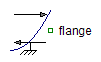Minimum - MapleSim Help

Minimum

Pass through the smallest signalDescription

The Minimum (or Min) component computes the minimum of the inputs.

The Use Vector boolean parameter selects the type of inputs.

 • If $\mathrm{false}$ (the default), the block has two scalar inputs, $\mathrm{u1}$ and $\mathrm{u2}$.
 • If $\mathrm{true}$, the block has a single vector input, $u$, and the Signal Size parameter sets the dimension of $u$. Signal Size must be greater than $1$.

Connections

 Name Description Modelica ID $\mathrm{u1}$ Real input signal 1 u1 $\mathrm{u2}$ Real input signal 2 u2 $y$ Real output signal y $u$ Real input vector u

Parameters

 Name Default Units Description Modelica ID Use Vector $\mathrm{false}$ True (checked) means use single input vector useVector Signal Size $2$ Dimension of the vector input signal (n>1) signalSize

 Modelica Standard Library The component described in this topic is from the Modelica Standard Library. To view the original documentation, which includes author and copyright information, click here.